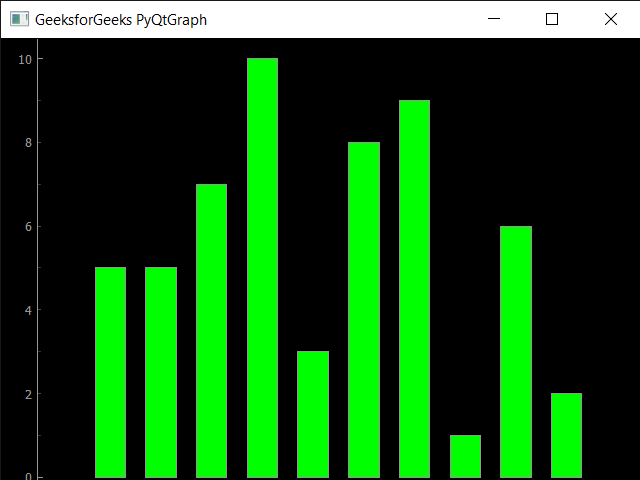GeeksforGeeks App
Open AppBrowser
Continue

# PyQtGraph – Getting Plot Item from Plot Window

In this article we will see how we can get the plot item of plot window in the PyQtGraph module. PyQtGraph is a graphics and user interface library for Python that provides functionality commonly required in designing and science applications. Its primary goals are to provide fast, interactive graphics for displaying data (plots, video, etc.) and second is to provide tools to aid in rapid application development (for example, property trees such as used in Qt Designer).Plot windows consist of two main parts: the Plot Panel containing the actual plotted graphics and the Control Panel. Plot item is basically the item which is plotted and is shown on the screen.

In order to do this we use getPlotItem method with the plot window object
Syntax : window.getPlotItem()
Argument : It takes no argument
Return : It returns PlotItem object

Below is the implementation

## Python3

 `# importing pyqtgraph as pg``import` `pyqtgraph as pg` `# importing QtCore and QtGui from the pyqtgraph module``from` `pyqtgraph.Qt ``import` `QtCore, QtGui` `# importing numpy as np``import` `numpy as np` `# define the data``title ``=` `"GeeksforGeeks PyQtGraph"` `# y values to plot by line 1``y ``=` `[``2``, ``8``, ``6``, ``8``, ``6``, ``11``, ``14``, ``13``, ``18``, ``19``]` `# y values to plot by line 2``y2 ``=` `[``3``, ``1``, ``5``, ``8``, ``9``, ``11``, ``16``, ``17``, ``14``, ``16``]``x ``=` `range``(``0``, ``10``)` `# create plot window object``plt ``=` `pg.plot()` `# showing x and y grids``plt.showGrid(x ``=` `True``, y ``=` `True``)` `# adding legend``plt.addLegend()` `# set properties of the label for y axis``plt.setLabel(``'left'``, ``'Vertical Values'``, units ``=``'y'``)` `# set properties of the label for x axis``plt.setLabel(``'bottom'``, ``'Horizontal Values'``, units ``=``'s'``)` `# setting horizontal range``plt.setXRange(``0``, ``10``)` `# setting vertical range``plt.setYRange(``0``, ``20``)` `# setting window title``plt.setWindowTitle(title)` `# plotting line in green color``line1 ``=` `plt.plot(x, y, pen ``=``'g'``, symbol ``=``'x'``, symbolPen ``=``'g'``, symbolBrush ``=` `0.2``, name ``=``'green'``)` `# plotting line2 with blue color``line2 ``=` `plt.plot(x, y2, pen ``=``'b'``, symbol ``=``'o'``, symbolPen ``=``'b'``, symbolBrush ``=` `0.2``, name ``=``'blue'``)` `# main method``if` `__name__ ``=``=` `'__main__'``:``    ` `    ``# importing system``    ``import` `sys``    ` `    ``# Start Qt event loop unless running in interactive mode or using``    ``if` `(sys.flags.interactive !``=` `1``) ``or` `not` `hasattr``(QtCore, ``'PYQT_VERSION'``):``        ``QtGui.QApplication.instance().exec_()`

Output :`Plot Item : pyqtgraph.graphicsItems.PlotItem.PlotItem.PlotItem object at 0x000002AF5282B8B0`

My Personal Notes arrow_drop_up